4.6 Exponential and logarithmic equations  (Page 7/8)

 Page 7 / 8

When does an extraneous solution occur? How can an extraneous solution be recognized?

When can the one-to-one property of logarithms be used to solve an equation? When can it not be used?

The one-to-one property can be used if both sides of the equation can be rewritten as a single logarithm with the same base. If so, the arguments can be set equal to each other, and the resulting equation can be solved algebraically. The one-to-one property cannot be used when each side of the equation cannot be rewritten as a single logarithm with the same base.

Algebraic

For the following exercises, use like bases to solve the exponential equation.

${4}^{-3v-2}={4}^{-v}$

$64\cdot {4}^{3x}=16$

$x=-\frac{1}{3}$

${3}^{2x+1}\cdot {3}^{x}=243$

${2}^{-3n}\cdot \frac{1}{4}={2}^{n+2}$

$n=-1$

$625\cdot {5}^{3x+3}=125$

$\frac{{36}^{3b}}{{36}^{2b}}={216}^{2-b}$

$b=\frac{6}{5}$

${\left(\frac{1}{64}\right)}^{3n}\cdot 8={2}^{6}$

For the following exercises, use logarithms to solve.

${9}^{x-10}=1$

$x=10$

$2{e}^{6x}=13$

${e}^{r+10}-10=-42$

No solution

$2\cdot {10}^{9a}=29$

$-8\cdot {10}^{p+7}-7=-24$

$p=\mathrm{log}\left(\frac{17}{8}\right)-7$

$7{e}^{3n-5}+5=-89$

${e}^{-3k}+6=44$

$k=-\frac{\mathrm{ln}\left(38\right)}{3}$

$-5{e}^{9x-8}-8=-62$

$-6{e}^{9x+8}+2=-74$

$x=\frac{\mathrm{ln}\left(\frac{38}{3}\right)-8}{9}$

${2}^{x+1}={5}^{2x-1}$

${e}^{2x}-{e}^{x}-132=0$

$7{e}^{8x+8}-5=-95$

$10{e}^{8x+3}+2=8$

$x=\frac{\mathrm{ln}\left(\frac{3}{5}\right)-3}{8}$

$4{e}^{3x+3}-7=53$

$8{e}^{-5x-2}-4=-90$

no solution

${3}^{2x+1}={7}^{x-2}$

${e}^{2x}-{e}^{x}-6=0$

$x=\mathrm{ln}\left(3\right)$

$3{e}^{3-3x}+6=-31$

For the following exercises, use the definition of a logarithm to rewrite the equation as an exponential equation.

$\mathrm{log}\left(\frac{1}{100}\right)=-2$

${10}^{-2}=\frac{1}{100}$

${\mathrm{log}}_{324}\left(18\right)=\frac{1}{2}$

For the following exercises, use the definition of a logarithm to solve the equation.

$5{\mathrm{log}}_{7}n=10$

$n=49$

$-8{\mathrm{log}}_{9}x=16$

$4+{\mathrm{log}}_{2}\left(9k\right)=2$

$k=\frac{1}{36}$

$2\mathrm{log}\left(8n+4\right)+6=10$

$10-4\mathrm{ln}\left(9-8x\right)=6$

$x=\frac{9-e}{8}$

For the following exercises, use the one-to-one property of logarithms to solve.

$\mathrm{ln}\left(10-3x\right)=\mathrm{ln}\left(-4x\right)$

${\mathrm{log}}_{13}\left(5n-2\right)={\mathrm{log}}_{13}\left(8-5n\right)$

$n=1$

$\mathrm{log}\left(x+3\right)-\mathrm{log}\left(x\right)=\mathrm{log}\left(74\right)$

$\mathrm{ln}\left(-3x\right)=\mathrm{ln}\left({x}^{2}-6x\right)$

No solution

${\mathrm{log}}_{4}\left(6-m\right)={\mathrm{log}}_{4}3m$

$\mathrm{ln}\left(x-2\right)-\mathrm{ln}\left(x\right)=\mathrm{ln}\left(54\right)$

No solution

${\mathrm{log}}_{9}\left(2{n}^{2}-14n\right)={\mathrm{log}}_{9}\left(-45+{n}^{2}\right)$

$\mathrm{ln}\left({x}^{2}-10\right)+\mathrm{ln}\left(9\right)=\mathrm{ln}\left(10\right)$

$x=±\frac{10}{3}$

For the following exercises, solve each equation for $\text{\hspace{0.17em}}x.$

$\mathrm{log}\left(x+12\right)=\mathrm{log}\left(x\right)+\mathrm{log}\left(12\right)$

$\mathrm{ln}\left(x\right)+\mathrm{ln}\left(x-3\right)=\mathrm{ln}\left(7x\right)$

$x=10$

${\mathrm{log}}_{2}\left(7x+6\right)=3$

$\mathrm{ln}\left(7\right)+\mathrm{ln}\left(2-4{x}^{2}\right)=\mathrm{ln}\left(14\right)$

$x=0$

${\mathrm{log}}_{8}\left(x+6\right)-{\mathrm{log}}_{8}\left(x\right)={\mathrm{log}}_{8}\left(58\right)$

$\mathrm{ln}\left(3\right)-\mathrm{ln}\left(3-3x\right)=\mathrm{ln}\left(4\right)$

$x=\frac{3}{4}$

${\mathrm{log}}_{3}\left(3x\right)-{\mathrm{log}}_{3}\left(6\right)={\mathrm{log}}_{3}\left(77\right)$

Graphical

For the following exercises, solve the equation for $\text{\hspace{0.17em}}x,$ if there is a solution . Then graph both sides of the equation, and observe the point of intersection (if it exists) to verify the solution.

${\mathrm{log}}_{9}\left(x\right)-5=-4$

$x=9$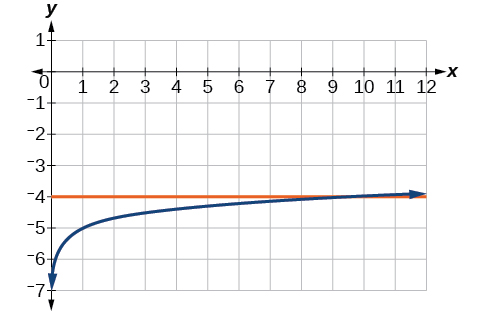${\mathrm{log}}_{3}\left(x\right)+3=2$

$\mathrm{ln}\left(3x\right)=2$

$x=\frac{{e}^{2}}{3}\approx 2.5$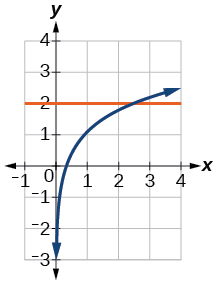$\mathrm{ln}\left(x-5\right)=1$

$\mathrm{log}\left(4\right)+\mathrm{log}\left(-5x\right)=2$

$x=-5$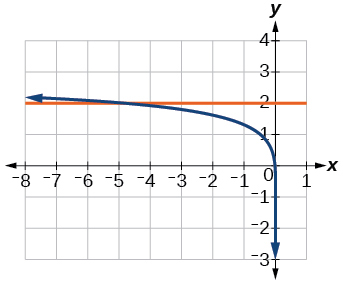$-7+{\mathrm{log}}_{3}\left(4-x\right)=-6$

$\mathrm{ln}\left(4x-10\right)-6=-5$

$x=\frac{e+10}{4}\approx 3.2$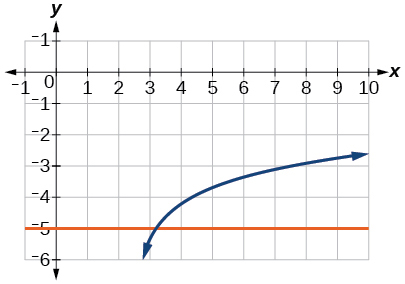$\mathrm{log}\left(4-2x\right)=\mathrm{log}\left(-4x\right)$

${\mathrm{log}}_{11}\left(-2{x}^{2}-7x\right)={\mathrm{log}}_{11}\left(x-2\right)$

No solution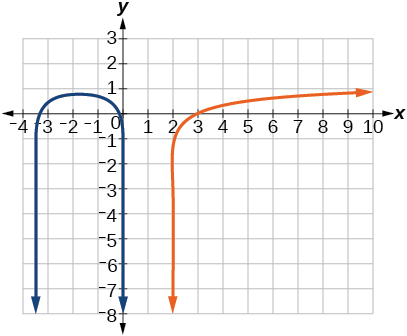$\mathrm{ln}\left(2x+9\right)=\mathrm{ln}\left(-5x\right)$

${\mathrm{log}}_{9}\left(3-x\right)={\mathrm{log}}_{9}\left(4x-8\right)$

$x=\frac{11}{5}\approx 2.2$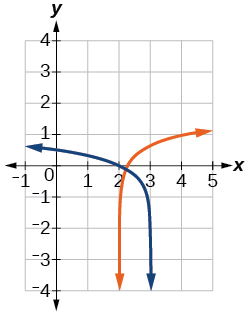$\mathrm{log}\left({x}^{2}+13\right)=\mathrm{log}\left(7x+3\right)$

$\frac{3}{{\mathrm{log}}_{2}\left(10\right)}-\mathrm{log}\left(x-9\right)=\mathrm{log}\left(44\right)$

$x=\frac{101}{11}\approx 9.2$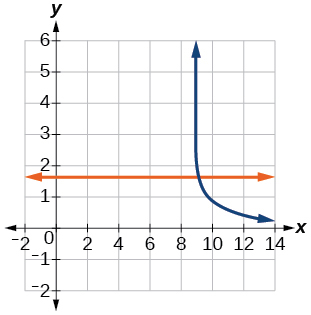$\mathrm{ln}\left(x\right)-\mathrm{ln}\left(x+3\right)=\mathrm{ln}\left(6\right)$

For the following exercises, solve for the indicated value, and graph the situation showing the solution point.

An account with an initial deposit of $\text{\hspace{0.17em}}\text{6,500}\text{\hspace{0.17em}}$ earns $\text{\hspace{0.17em}}7.25%\text{\hspace{0.17em}}$ annual interest, compounded continuously. How much will the account be worth after 20 years?

about $\text{\hspace{0.17em}}27,710.24$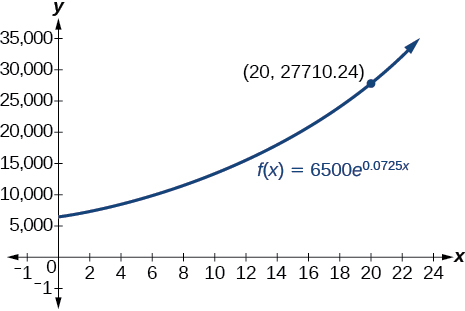The formula for measuring sound intensity in decibels $\text{\hspace{0.17em}}D\text{\hspace{0.17em}}$ is defined by the equation $\text{\hspace{0.17em}}D=10\mathrm{log}\left(\frac{I}{{I}_{0}}\right),\text{}$ where $\text{\hspace{0.17em}}I\text{\hspace{0.17em}}$ is the intensity of the sound in watts per square meter and $\text{\hspace{0.17em}}{I}_{0}={10}^{-12}\text{\hspace{0.17em}}$ is the lowest level of sound that the average person can hear. How many decibels are emitted from a jet plane with a sound intensity of $\text{\hspace{0.17em}}8.3\cdot {10}^{2}\text{\hspace{0.17em}}$ watts per square meter?

The population of a small town is modeled by the equation $\text{\hspace{0.17em}}P=1650{e}^{0.5t}\text{\hspace{0.17em}}$ where $\text{\hspace{0.17em}}t\text{\hspace{0.17em}}$ is measured in years. In approximately how many years will the town’s population reach $\text{\hspace{0.17em}}\text{20,000?}$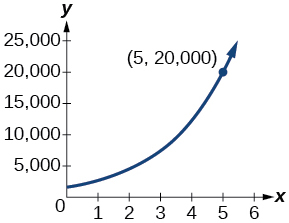Technology

For the following exercises, solve each equation by rewriting the exponential expression using the indicated logarithm. Then use a calculator to approximate $\text{\hspace{0.17em}}x\text{\hspace{0.17em}}$ to 3 decimal places .

$1000{\left(1.03\right)}^{t}=5000\text{\hspace{0.17em}}$ using the common log.

${e}^{5x}=17\text{\hspace{0.17em}}$ using the natural log

$\frac{\mathrm{ln}\left(17\right)}{5}\approx 0.567$

$3{\left(1.04\right)}^{3t}=8\text{\hspace{0.17em}}$ using the common log

${3}^{4x-5}=38\text{\hspace{0.17em}}$ using the common log

$50{e}^{-0.12t}=10\text{\hspace{0.17em}}$ using the natural log

For the following exercises, use a calculator to solve the equation. Unless indicated otherwise, round all answers to the nearest ten-thousandth.

$7{e}^{3x-5}+7.9=47$

$x\approx 2.2401$

$\mathrm{ln}\left(3\right)+\mathrm{ln}\left(4.4x+6.8\right)=2$

$\mathrm{log}\left(-0.7x-9\right)=1+5\mathrm{log}\left(5\right)$

$x\approx -\text{44655}.\text{7143}$

Atmospheric pressure $\text{\hspace{0.17em}}P\text{\hspace{0.17em}}$ in pounds per square inch is represented by the formula $\text{\hspace{0.17em}}P=14.7{e}^{-0.21x},$ where $x$ is the number of miles above sea level. To the nearest foot, how high is the peak of a mountain with an atmospheric pressure of $\text{\hspace{0.17em}}8.369\text{\hspace{0.17em}}$ pounds per square inch? ( Hint : there are 5280 feet in a mile)

The magnitude M of an earthquake is represented by the equation $\text{\hspace{0.17em}}M=\frac{2}{3}\mathrm{log}\left(\frac{E}{{E}_{0}}\right)\text{\hspace{0.17em}}$ where $\text{\hspace{0.17em}}E\text{\hspace{0.17em}}$ is the amount of energy released by the earthquake in joules and $\text{\hspace{0.17em}}{E}_{0}={10}^{4.4}\text{\hspace{0.17em}}$ is the assigned minimal measure released by an earthquake. To the nearest hundredth, what would the magnitude be of an earthquake releasing $\text{\hspace{0.17em}}1.4\cdot {10}^{13}\text{\hspace{0.17em}}$ joules of energy?

about $\text{\hspace{0.17em}}5.83$

Extensions

Use the definition of a logarithm along with the one-to-one property of logarithms to prove that $\text{\hspace{0.17em}}{b}^{{\mathrm{log}}_{b}x}=x.$

Recall the formula for continually compounding interest, $\text{\hspace{0.17em}}y=A{e}^{kt}.\text{\hspace{0.17em}}$ Use the definition of a logarithm along with properties of logarithms to solve the formula for time $\text{\hspace{0.17em}}t\text{\hspace{0.17em}}$ such that $\text{\hspace{0.17em}}t\text{\hspace{0.17em}}$ is equal to a single logarithm.

$t=\mathrm{ln}\left({\left(\frac{y}{A}\right)}^{\frac{1}{k}}\right)$

Recall the compound interest formula $\text{\hspace{0.17em}}A=a{\left(1+\frac{r}{k}\right)}^{kt}.\text{\hspace{0.17em}}$ Use the definition of a logarithm along with properties of logarithms to solve the formula for time $\text{\hspace{0.17em}}t.$

Newton’s Law of Cooling states that the temperature $\text{\hspace{0.17em}}T\text{\hspace{0.17em}}$ of an object at any time t can be described by the equation $\text{\hspace{0.17em}}T={T}_{s}+\left({T}_{0}-{T}_{s}\right){e}^{-kt},$ where $\text{\hspace{0.17em}}{T}_{s}\text{\hspace{0.17em}}$ is the temperature of the surrounding environment, $\text{\hspace{0.17em}}{T}_{0}\text{\hspace{0.17em}}$ is the initial temperature of the object, and $\text{\hspace{0.17em}}k\text{}$ is the cooling rate. Use the definition of a logarithm along with properties of logarithms to solve the formula for time $\text{\hspace{0.17em}}t\text{\hspace{0.17em}}$ such that $\text{\hspace{0.17em}}t\text{\hspace{0.17em}}$ is equal to a single logarithm.

$t=\mathrm{ln}\left({\left(\frac{T-{T}_{s}}{{T}_{0}-{T}_{s}}\right)}^{-\text{\hspace{0.17em}}\frac{1}{k}}\right)$

find the equation of the line if m=3, and b=-2
graph the following linear equation using intercepts method. 2x+y=4
Ashley
how
Wargod
what?
John
ok, one moment
UriEl
how do I post your graph for you?
UriEl
it won't let me send an image?
UriEl
also for the first one... y=mx+b so.... y=3x-2
UriEl
y=mx+b you were already given the 'm' and 'b'. so.. y=3x-2
Tommy
"7"has an open circle and "10"has a filled in circle who can I have a set builder notation
x=-b+_Гb2-(4ac) ______________ 2a
I've run into this: x = r*cos(angle1 + angle2) Which expands to: x = r(cos(angle1)*cos(angle2) - sin(angle1)*sin(angle2)) The r value confuses me here, because distributing it makes: (r*cos(angle2))(cos(angle1) - (r*sin(angle2))(sin(angle1)) How does this make sense? Why does the r distribute once
so good
abdikarin
this is an identity when 2 adding two angles within a cosine. it's called the cosine sum formula. there is also a different formula when cosine has an angle minus another angle it's called the sum and difference formulas and they are under any list of trig identities
strategies to form the general term
carlmark
How can you tell what type of parent function a graph is ?
generally by how the graph looks and understanding what the base parent functions look like and perform on a graph
William
if you have a graphed line, you can have an idea by how the directions of the line turns, i.e. negative, positive, zero
William
y=x will obviously be a straight line with a zero slope
William
y=x^2 will have a parabolic line opening to positive infinity on both sides of the y axis vice versa with y=-x^2 you'll have both ends of the parabolic line pointing downward heading to negative infinity on both sides of the y axis
William
y=x will be a straight line, but it will have a slope of one. Remember, if y=1 then x=1, so for every unit you rise you move over positively one unit. To get a straight line with a slope of 0, set y=1 or any integer.
Aaron
yes, correction on my end, I meant slope of 1 instead of slope of 0
William
what is f(x)=
I don't understand
Joe
Typically a function 'f' will take 'x' as input, and produce 'y' as output. As 'f(x)=y'. According to Google, "The range of a function is the complete set of all possible resulting values of the dependent variable (y, usually), after we have substituted the domain."
Thomas
Sorry, I don't know where the "Â"s came from. They shouldn't be there. Just ignore them. :-)
Thomas
Darius
Thanks.
Thomas
Â
Thomas
It is the Â that should not be there. It doesn't seem to show if encloses in quotation marks. "Â" or 'Â' ... Â
Thomas
Now it shows, go figure?
Thomas
what is this?
i do not understand anything
unknown
lol...it gets better
Darius
I've been struggling so much through all of this. my final is in four weeks 😭
Tiffany
this book is an excellent resource! have you guys ever looked at the online tutoring? there's one that is called "That Tutor Guy" and he goes over a lot of the concepts
Darius
thank you I have heard of him. I should check him out.
Tiffany
is there any question in particular?
Joe
I have always struggled with math. I get lost really easy, if you have any advice for that, it would help tremendously.
Tiffany
Sure, are you in high school or college?
Darius
Hi, apologies for the delayed response. I'm in college.
Tiffany
how to solve polynomial using a calculator
So a horizontal compression by factor of 1/2 is the same as a horizontal stretch by a factor of 2, right?
The center is at (3,4) a focus is at (3,-1), and the lenght of the major axis is 26
The center is at (3,4) a focus is at (3,-1) and the lenght of the major axis is 26 what will be the answer?
Rima
I done know
Joe
What kind of answer is that😑?
Rima
I had just woken up when i got this message
Joe
Can you please help me. Tomorrow is the deadline of my assignment then I don't know how to solve that
Rima
i have a question.
Abdul
how do you find the real and complex roots of a polynomial?
Abdul
@abdul with delta maybe which is b(square)-4ac=result then the 1st root -b-radical delta over 2a and the 2nd root -b+radical delta over 2a. I am not sure if this was your question but check it up
Nare
This is the actual question: Find all roots(real and complex) of the polynomial f(x)=6x^3 + x^2 - 4x + 1
Abdul
@Nare please let me know if you can solve it.
Abdul
I have a question
juweeriya
hello guys I'm new here? will you happy with me
mustapha
The average annual population increase of a pack of wolves is 25.
how do you find the period of a sine graph
Period =2π if there is a coefficient (b), just divide the coefficient by 2π to get the new period
Am
if not then how would I find it from a graph
Imani
by looking at the graph, find the distance between two consecutive maximum points (the highest points of the wave). so if the top of one wave is at point A (1,2) and the next top of the wave is at point B (6,2), then the period is 5, the difference of the x-coordinates.
Am
you could also do it with two consecutive minimum points or x-intercepts
Am
I will try that thank u
Imani
Case of Equilateral Hyperbola
ok
Zander
ok
Shella
f(x)=4x+2, find f(3)
Benetta
f(3)=4(3)+2 f(3)=14
lamoussa
14
Vedant
pre calc teacher: "Plug in Plug in...smell's good" f(x)=14
Devante
8x=40
Chris
Explain why log a x is not defined for a < 0
the sum of any two linear polynomial is whatByByByByBy David Martin